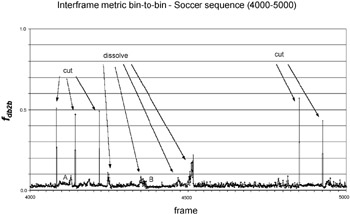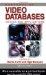# 3. A MLP-Based Technique

## 3. A MLP-Based Technique

As already stated, one of the critical aspects of most of the techniques discussed in previous section is the determination of thresholds or, in general, the definition of criteria of detection and classification of the transitions. Moreover, most of the techniques present in literature are strongly dependent on the kind of sequences analyzed. To cope with these problems we propose the use of a neural network that analyzes the sequence of interframe metric values and is able to detect shot transitions, also producing a coarse classification of the detected transitions. This approach may be considered a generalization of the MLP-based algorithm already proposed in .

### 3.1 Short Notes on Neural Networks

In the last decades, neural networks  have been successfully used in many problems of pattern recognition and classification. Briefly, a neural network is a set of units or nodes connected by links or synapses. A numeric weight is associated to each link; the set of weights represents the memory of the network, where knowledge is stored. The determination of these weights is done during the learning phase. There are three basic classes of learning paradigms : supervised learning (i.e., performed under an external supervision), reinforcement learning (i.e., through a trial-and-error process) and unsupervised learning (i.e., performed in a self-organized manner).

The network interacts with the environment in which it is embedded through a set of input nodes and a set of output nodes, respectively. During the learning process, synaptic weights are modified in an orderly fashion so as input-output pairs fit a desired function. Each processing unit is characterized by a set of connecting links to other units, a current activation level and an activation function used to determine the activation level in the next step, given the input weights.

A multilayer perceptron or MLP exhibits a network architecture of the kind shown in Figure 7.5. It is a multilayer (i.e., the network units are organized in the form of layers) feedforward (i.e., signals propagate through the network in a forward direction) neural network characterized by the presence of one or more hidden layers, whose nodes are correspondingly called hidden units. This network architecture, already proposed in the fifties, has been applied successfully to solve diverse problems after the introduction ,  of the highly popular learning algorithm known as error back-propagation algorithm. This supervised learning algorithm is based on the error-correction learning rule.Figure 7.5: An example of multilayer perceptron with one hidden layer.

Basically, the back-propagation process consists of two passes through the different network layers, a forward pass and a backward pass. In the forward pass, an input vector (training pattern) is applied to the input nodes, and its effect propagates through the network, layer by layer, so as to produce a set of outputs as the actual response of the network. In this phase the synaptic weights are all fixed. During the backward pass, the synaptic weights are all adjusted in accordance with the error-correction rule. Specifically, the actual response of the network is subtracted from the desired response to produce an error signal. This error signal is then propagated backward through the network, and the synaptic weights are adjusted so as to make the actual response of the network move closer to the desired response. The process is then iterated until the synaptic weights stabilize and the error converges to some minimum, or acceptably small, value. In practical applications, learning results from the many presentations of a prescribed set of training examples to the network. One complete presentation of the entire training set is called an epoch. It is common practice to randomize the order of presentation of training examples from one epoch to the next.

### 3.2 Use of MLPs in Temporal Segmentation

We propose the use of a MLP with an input layer, an hidden layer and an output layer, whose input vector is a set of interframe metric difference values. The training set is made up by examples extracted from sequences containing abrupt transitions, gradual transitions or no transition at all. We adopted the bin-to-bin luminance histogram difference as an interframe metric. This choice is due to the simpleness of this metric, to its sufficient representativity with respect to both abrupt and gradual transitions, and to its ability to provide a simple interpretation model of the general video content evolution. As an example, Figure 7.6 illustrates the evolution of the bin-to-bin metric within a frame interval of 1000 frames, extracted from a soccer video sequence. Five cuts and four dissolves are present in the sequence, and all these shot boundaries are clearly present in the figure. In the same figure, it is also evident as gradual transitions are sometimes very difficult to distinguish from intrashot sequences (e.g., compare A and B in the figure).Figure 7.6: Interframe bin-to-bin metric for a soccer sequence.

The MLP's input units are fed with the values of the HDM computed within a temporal window of several frames (the details about the choice of the number of input and hidden units will be provided in the next section). The output units are three, each of them representing one of three distinct possibilities: abrupt transition, gradual transition or no break at all. Output units assume real values in the range 0 - 1. Table 7.1 shows the output values used during the training process, in correspondence to the following input configurations: abrupt transition at the center of the temporal window, gradual transition with its maximum located near the center of the temporal window, no-transition.

Table 7.1: Output values of the MLP during the training phase

O1

O2

O3

Abrupt transition

0.99

0.01

0.01

Gradual transition

0.01

0.99

0.01

No transition

0.01

0.01

0.99

During the learning phase, the position of each transition in the input video sequence is known, thus defining the correct triple of output values for each position of the input temporal window, i.e., for each input pattern in the training set. The above described back-propagation process therefore allows for the weights adjustment. When the trained network analyzes an unknown input sequence, at each step of the analysis the highest value of the output triple determines the detection of an abrupt or gradual transition, or the absence of transitions, in the analyzed temporal window. As a consequence, explicit threshold values are not required for the decision.Handbook of Video Databases: Design and Applications (Internet and Communications)
ISBN: 084937006X
EAN: 2147483647
Year: 2003
Pages: 393

flylib.com © 2008-2017.
If you may any questions please contact us: flylib@qtcs.net